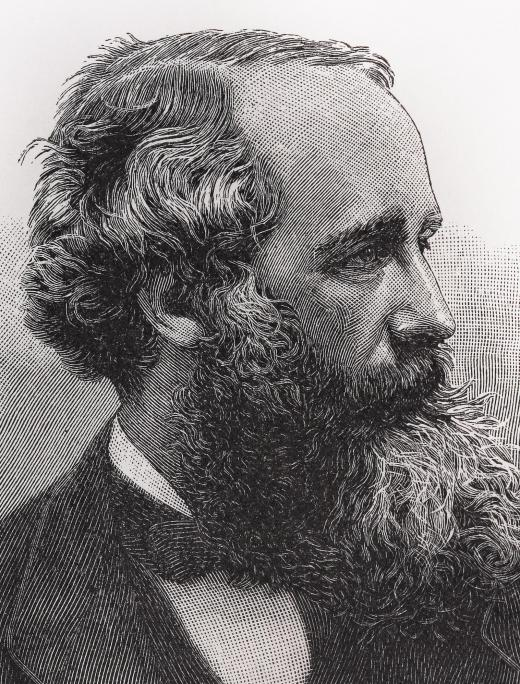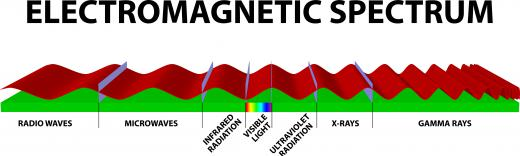Science
Fact Checked

# What is Electromagnetic Wave Theory?

Jason C. Chavis
Jason C. Chavis

The concept known as electromagnetic wave theory originated with the work of James Clerk Maxwell and Heinrich Hertz. According to the electric and magnetic equations postulated by Maxwell, electromagnetic fields resemble a wave in both structure and action. Electromagnetic waves coincide with the measurement of the speed of light, making light an electromagnetic wave itself.

Electric fields are spatially-varying and generate a magnetic field that is time-varying. Likewise, magnetic fields will do the same for electric fields, making the two concepts operate in unison. Together the two fields will oscillate and create an electromagnetic wave.

The physical properties of the electromagnetic wave theory take the form of electrodynamics. This facet of the theory means that any electromagnetic field present in the same space is considered a vector field, a wave with direction and length. As such, it can merge together with other vector fields. For example, when an electromagnetic wave impacts a molecule, the atoms within that molecule begin to oscillate, emitting their own electromagnetic waves, impacting the original wave. According to electromagnetic wave theory, this will cause refraction, a change in velocity, or diffraction, a change in wavelength.Physicist James Clerk Maxwell was noted for his work with electromagnetism.

Since light is a type of electromagnetic wave, the theory determines that the oscillation of light cannot be affected by other static electric or magnetic fields. However, interactions between certain exterior events, such as light traveling through a crystal, can have an effect. According to electromagnetic wave theory, magnetic fields impacting light will cause the Faraday effect and electric fields impacting light will cause the Kerr effect, a reduction of the speed of light waves.When an electromagnetic wave creates waves at different frequencies, it is considered a spectrum.

Frequency is a very important aspect of this theory. Oscillation of the wave is measured by hertz, the unit for frequency. One hertz is equal to one oscillation per second. When an electromagnetic wave, as in the case of light, creates waves at different frequencies, it is considered a spectrum.

Small particles of energy called photons are the basic units of electromagnetic radiation. As the photons travel, the wave follows and creates a frequency proportional to the particle. Photons are absorbed by atoms, which, in turn, excite electrons. When the electron reaches a high enough energy level, it escapes the positive attraction of the nucleus. If the electron energy level lowers, a photon of light is emitted.

The electromagnetic wave theory states that any acceleration of an electric charge or change in the magnetic field produces radiation. This radiation can come in the form of either a wave or a particle. Velocity, wavelength and frequency are the factors associated with waves. Particles contain individualized energy equal to the frequency. Regardless of the type, electromagnetic radiation travels at the speed of light in a vacuum. This fact prompted Albert Einstein to establish the theory of relativity.

## You might also Like